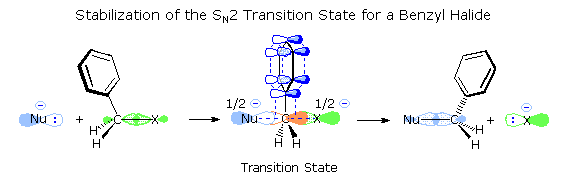# What is the order of increasing "S"_text(N)2 activity of the following compounds?

## $\text{CH"_3"CH"_2"X}$ $\text{PhCH"_2"X}$ ("CH"_3)_2"CHX" $\text{CH"_2"=CHCH"_2"X}$

Jan 18, 2017

("CH"_3)_2"-CHX" < "CH"_3"-CH"_2"X" < "CH"_2"=CH-CH"_2"X" < "Ph-CH"_2"X"

#### Explanation:

The $\text{S"_"N} 2$ reaction involves the backside attack of a nucleophile on a substrate at the same time as the leaving group departs.The rate of the reaction depends on steric and mesomeric effects.

The mesomeric effect causes allylic and benzylic halides to have faster $\text{S"_"N} 2$ rates than alkyl halides.

This is because the π-electrons can overlap with the partially broken and formed bonds in the transition state.

This lowers the activation energy and increases the reaction rate.The phenyl group can delocalize the partial charge better than a vinyl group can.

The extra methyl group in isopropyl halides (on the right) makes it more sterically hindered.("CH"_3)_2"-CHX" < "CH"_3"-CH"_2"X" < "CH"_2"=CH-CH"_2"X" < "Ph-CH"_2"X"